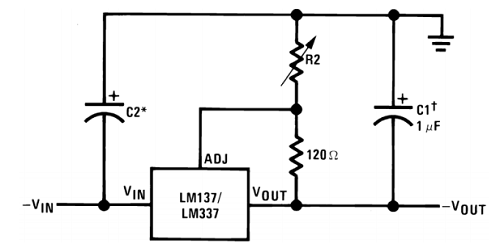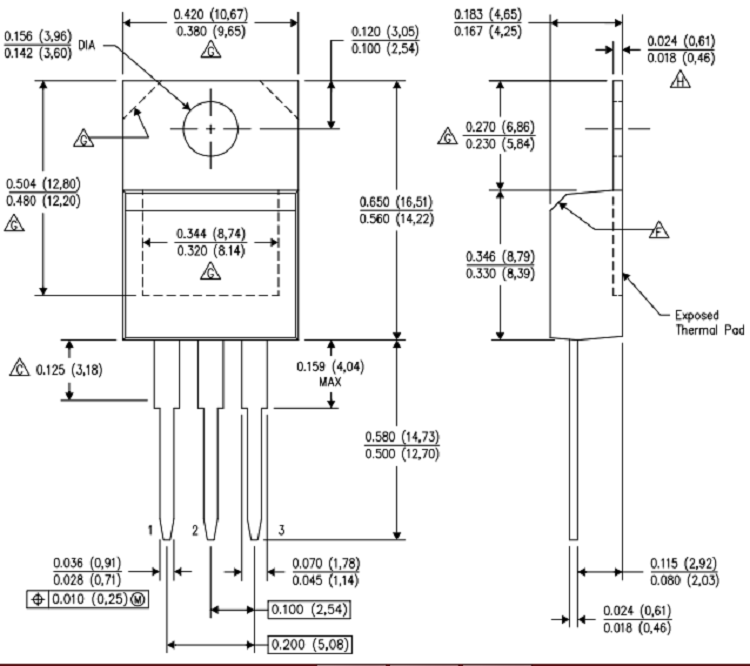LM337 Variable Negative Voltage Regulator

The LM337 is a Variable Negative Voltage Regulator that can output voltage from -1.25V to -37V with a maximum output current of -1.5A.

LM337 Pin Configuration

 Pin Number Pin Name Description 1 Adjust This pins adjusts the output voltage 2 Input Voltage (Vin) The input voltage which has to be regulated is given to this pin 3 Output Voltage (Vout) The regulated output voltage set by the adjust pin can be obtained from this pin

Features

• Adjustable 3-terminal Negative voltage regulator
• Output voltage can be set to range from -1.25V to -37V
• Maximum Output current is -1.5A
• Differential Input and Output Voltage is 40V(max), recommended 15V
• Maximum output current when voltage difference is 15V is -2.2A
• Operating junction temperature is 125°C
• Available in To-220, SOT223, TO263 Package

Note: Complete Technical Details can be found at the LM337 datasheet provided at the end of this page.

Alternative Negative Voltage Regulators

LM137, LM7909, LM7905, LM7912

LM337 Equivalents

LT137, PB137, LM317 (Positive Variable Voltage regulator)

LM337 Voltage Regulator Description

The LM337 is the equivalent counterpart of the LM317 Positive Voltage regulator. The IC commonly used in Adjustable Lab Voltage generators in combination with the LM317 IC. It can also be used as Current Regulators and negative voltage protection circuits, refer the datasheet linked below for application circuits.

The IC has three pins, in which the input voltage is supplied to VIN pin then using a pair of resistors (potential divider) we set a voltage at Adjust pin which will decide the output voltage of the IC that is given out at VOUT pin. Now to make it act as a variable voltage regulator we have to set variable voltages which can be done by using a potentiometer in the potential divider (R2 shown below). The below circuit is shown for reference from the LM337 datasheet.The output voltage thus obtained can be calculated using the below formulae

VOUT = - 1.25 × (1 + (R2/R1))

The actual formulae in the datasheet will also include Iadj (Current through the adjust pin), but for simplification purpose we have assumed Iadj to be 50uA constant which will slightly differ in practical application.

Also note that the output current of the IC will not be 1.5A always, if the difference voltage between the input and output voltage is high the output current will also decrease. Refer to the difference voltage vs Output Current graph from the datasheet for more information.

Applications

• Used for Positive voltage regulations
• Variable power supply
• Current limiting circuits
• Reverse polarity circuits
• Commonly used in Desktop PC, DVD and other consumer products
• Used in motor control circuits

2D – Model of LM337 (TO-220)Component Datasheet

Related Post

Join 20K+subscribers

We will never spam you.

* indicates required

Be a part of our ever growing community.